• 相关性检验 本文给出两种相关系数，系数越大说明越相关。你可能会参考另一篇博客独立性检验。 皮尔森相关系数 皮尔森相关系数（Pearson correlation coefficient）也叫皮尔森积差相关系数（Pearson product...
相关性检验

本文给出两种相关系数，系数越大说明越相关。你可能会参考另一篇博客独立性检验。
皮尔森相关系数
皮尔森相关系数（Pearson correlation coefficient）也叫皮尔森积差相关系数（Pearson product-moment correlation coefficient），是用来反应两个变量相似程度的统计量。或者说可以用来计算两个向量的相似度（在基于向量空间模型的文本分类、用户喜好推荐系统中都有应用）。
皮尔森相关系数计算公式如下：

分子是协方差，分子是两个变量标准差的乘积。显然要求X和Y的标准差都不能为0。
因为,所以皮尔森相关系数计算公式还可以写成：

当两个变量的线性关系增强时，相关系数趋于1或-1。正相关时趋于1，负相关时趋于-1。当两个变量独立时相关系统为0，但反之不成立。比如对于，X服从[-1,1]上的均匀分布，此时E(XY)为0，E(X)也为0，所以，但x和y明显不独立。所以“不相关”和“独立”是两回事。当Y 和X服从联合正态分布时，其相互独立和不相关是等价的。
对于居中的数据来说（何谓居中？也就是每个数据减去样本均值，居中后它们的平均值就为0），E(X)=E(Y)=0，此时有：
即相关系数可以看作是两个随机变量中得到的样本集向量之间夹角的cosine函数。
进一步当X和Y向量归一化后，||X||=||Y||=1，相关系数即为两个向量的乘积。
Spearman秩相关系数
首先说明秩相关系数还有其他类型，比如kendal秩相关系数。
使用Pearson线性相关系数有2个局限：
必须假设数据是成对地从正态分布中取得的。
数据至少在逻辑范围内是等距的。
对于更一般的情况有其他的一些解决方案，Spearman秩相关系数就是其中一种。Spearman秩相关系数是一种无参数（与分布无关）检验方法，用于度量变量之间联系的强弱。在没有重复数据的情况下，如果一个变量是另外一个变量的严格单调函数，则Spearman秩相关系数就是+1或-1，称变量完全Spearman秩相关。注意这和Pearson完全相关的区别，只有当两变量存在线性关系时，Pearson相关系数才为+1或-1。
对原始数据xi,yi按从大到小排序，记x'i,y'i为原始xi,yi在排序后列表中的位置，x'i,y'i称为xi,yi的秩次，秩次差di=x'i-y'i。Spearman秩相关系数为：

位置
原始X
排序后
秩次
原始Y
排序后
秩次
秩次差
1
12
546
5
1
78
6
1
2
546
45
1
78
46
1
0
3
13
32
4
2
45
5
1
4
45
13
2
46
6
2
0
5
32
12
3
6
2
4
1
6
2
2
6
45
1
3
-3
对于上表数据，算出Spearman秩相关系数为：1-6*(1+1+1+9)/(6*35)=0.6571
查阅秩相关系数检验的临界值表
n
显著水平
0.01
0.05
5
0.9
1
6
0.829
0.943
7
0.714
0.893
n=6时，0.6571<0.829，所以在0.01的显著水平下认为X和Y是不相关的。
如何原始数据中有重复值，则在求秩次时要以它们的平均值为准，比如：
原始X
秩次
调整后的秩次
0.8
5
5
1.2
4
(4+3)/2=3.5
1.2
3
(4+3)/2=3.5
2.3
2
2
18
1
1
Spearman秩相关系数应该是从秩和检验延伸过来的，因为它们很像。

转载于:https://www.cnblogs.com/leaf9/archive/2013/04/09/3009931.html
展开全文• ## 详解相关性检验

千次阅读 2020-02-09 21:16:10
相关性检验 使用皮尔逊相关系数和斯皮尔曼相关系数来衡量两个变量间相关性的大小 文章目录(1)描述性统计1.使用Matlab进行计算2.使用Excel计算3.使用Spss计算(2)正态分布的检验0.偏度与峰度1. JB 检验(大样本n>...
相关性检验
使用皮尔逊相关系数和斯皮尔曼相关系数来衡量两个变量间相关性的大小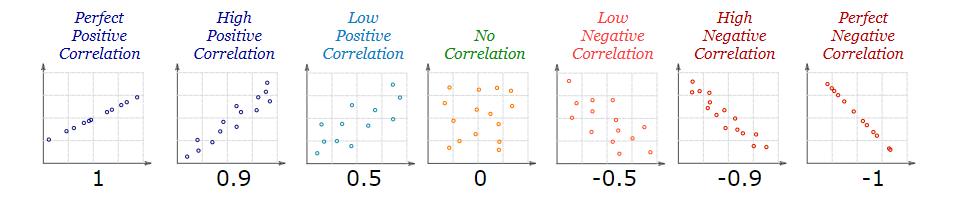文章目录(1)描述性统计1.使用Matlab进行计算2.使用Excel计算3.使用Spss计算(2)正态分布的检验0.偏度与峰度1. JB 检验(大样本n>30)2. 夏皮洛-威尔克检验 (3)皮尔逊相关系数1.总体皮尔逊Person相关系数2.样本皮尔逊Person相关系数3.使用皮尔逊Person相关系数的注意事项4.使用matlab计算person系数(3)对皮尔逊相关系数进行假设检验1.可以进行假设检验的前提2.进行正态分布检验3.流程(4)斯皮尔曼(sperman)相关系数1. 斯皮尔曼相关系数的第一种定义2. 斯皮尔曼相关系数的第二种定义3.使用matlab计算斯皮尔曼相关系数(5)斯皮尔曼相关系数的假设检验1.小样本情况下$(n\le30)$2.大样本情况下$(n\ge30)$(6)person与spearman对比
(1)描述性统计

所谓描述性统计，就是对已有的数据的多个特征(最小元素，最大元素，均值，中位数等)进行计算。

1.使用Matlab进行计算

min求最小值
max求最大值
mean求平均值
median求中位数的值
skewness求偏度
kurtosis求峰度
std求标准差

2.使用Excel计算3.使用Spss计算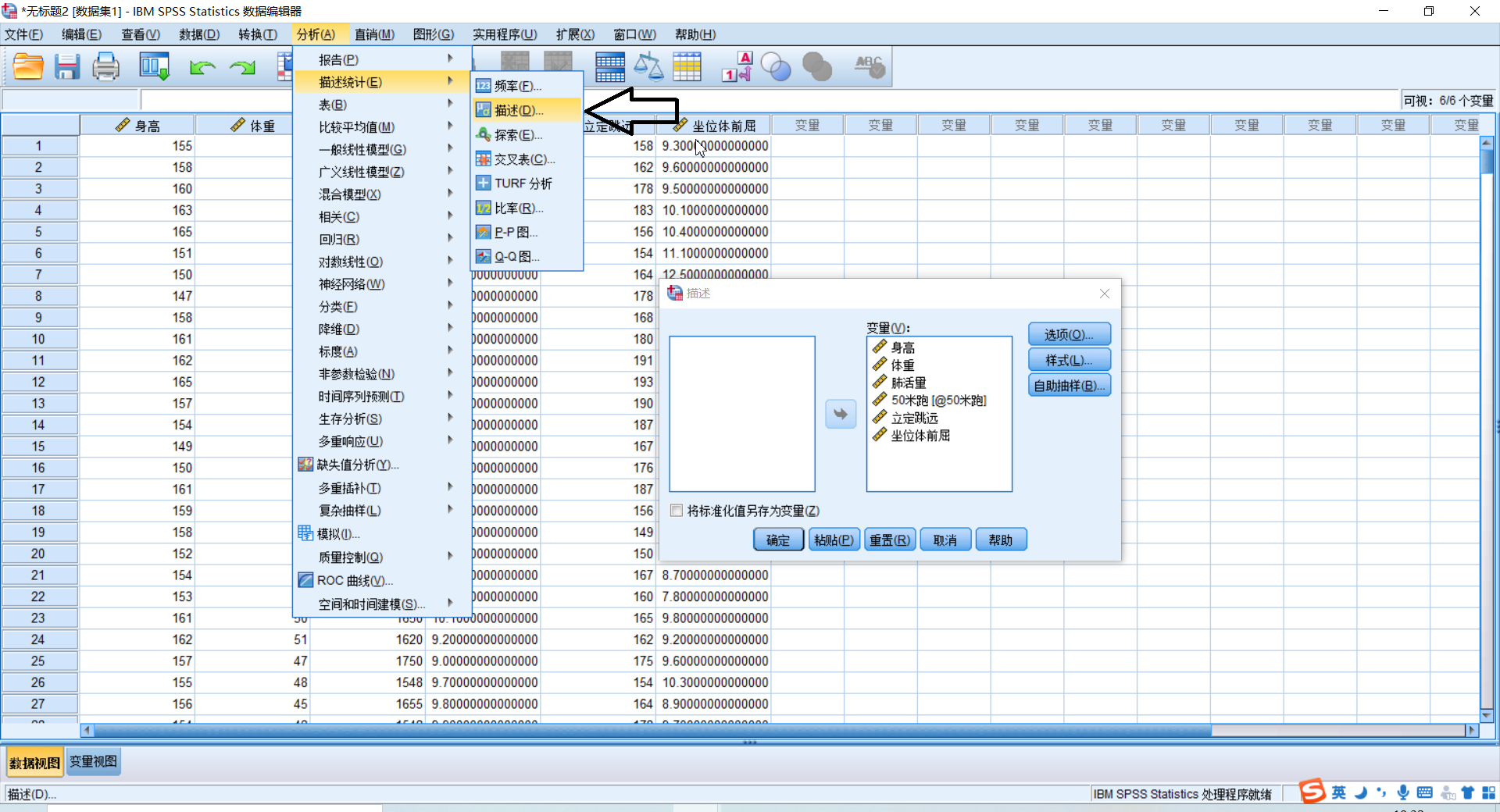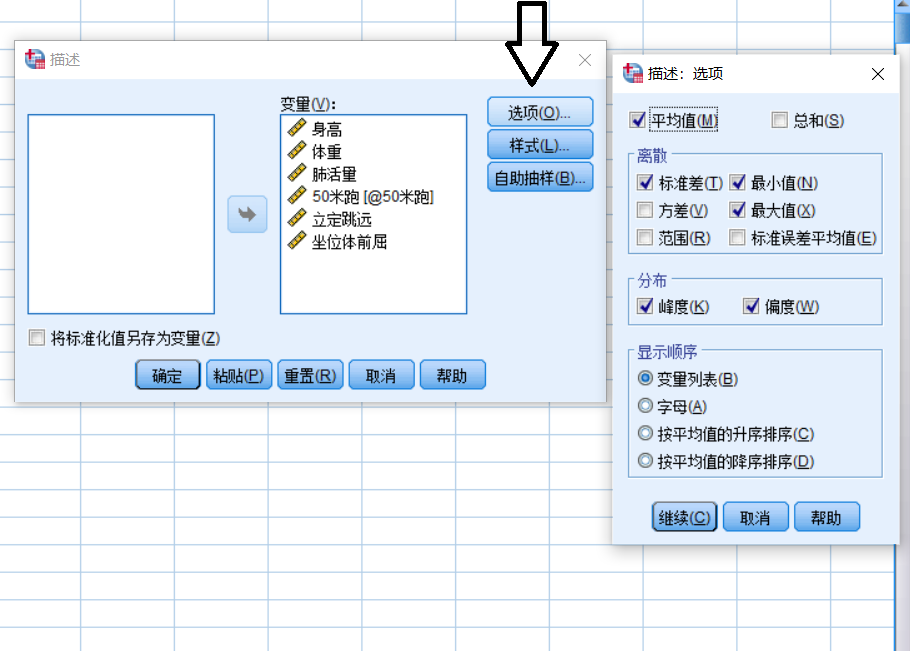(2)正态分布的检验
0.偏度与峰度

偏度：是统计数据分布偏斜方向和程度的度量，是统计数据分布非对称程度的数字特征。定义上偏度是样本的三阶标准化矩。
$S=E[(\dfrac{X-\mu}{\sigma})^3]$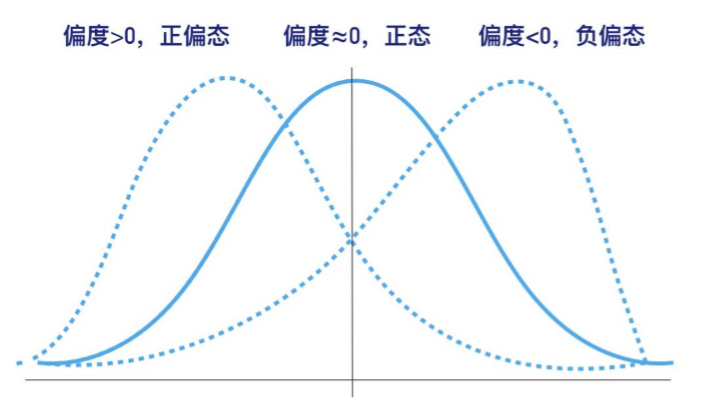注意正态分布的偏度为0

峰度：表征概率密度分布曲线在平均值处峰值高低的特征数。直观看来，峰度反映了峰部的尖度。定义上偏度是样本的四阶标准化矩。
$K=E[(\dfrac{X-\mu}{\sigma})^4]$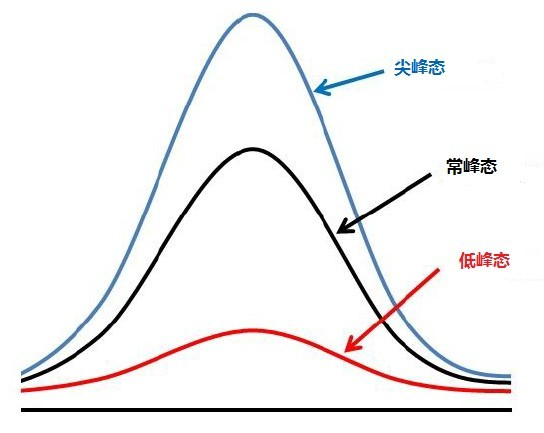注意正态分布的峰度为3
1. JB 检验(大样本n>30)

step 1：进行假设检验 $H_0:$该变量服从正态分布，$H_1:$该变量不服从正态分布。
step 2：假设对一个随机变量 $X_i$ ，其峰度为 $K$ ，其偏度为 $S$，则构造检验统计量$JB=\dfrac{n}{6}[S^2+\dfrac{(K-3)^2}{4}]\sim \chi^2(2)$服从自由度为2的卡方分布。
step 3： 代入数据计算出 $JB^*$，通过$JB^*$计算出对应的 $p$ 值。
step 4：通过 $p$ 值检验得出结论。(一般取 $p$ 为 $0.05$ )。
matlab中：[h,p]=jbtest(x,alpha),$x$ 表示要检测的变量，只能取向量，alpha表示显著性水平，h=1表示拒绝原假设，h=0表示接受原假设，p表示返回的p值。

2. 夏皮洛-威尔克检验

step 1：进行假设检验 $H_0:$该变量服从正态分布，$H_1:$该变量不服从正态分布。
step 2：使用SPSS计算出相应的 $p$ 值。
step 3：通过相应的 $p$ 值进行判断。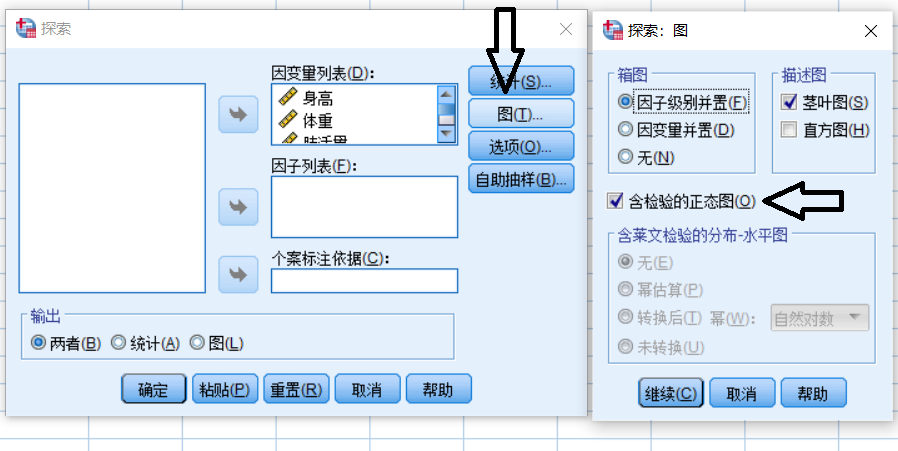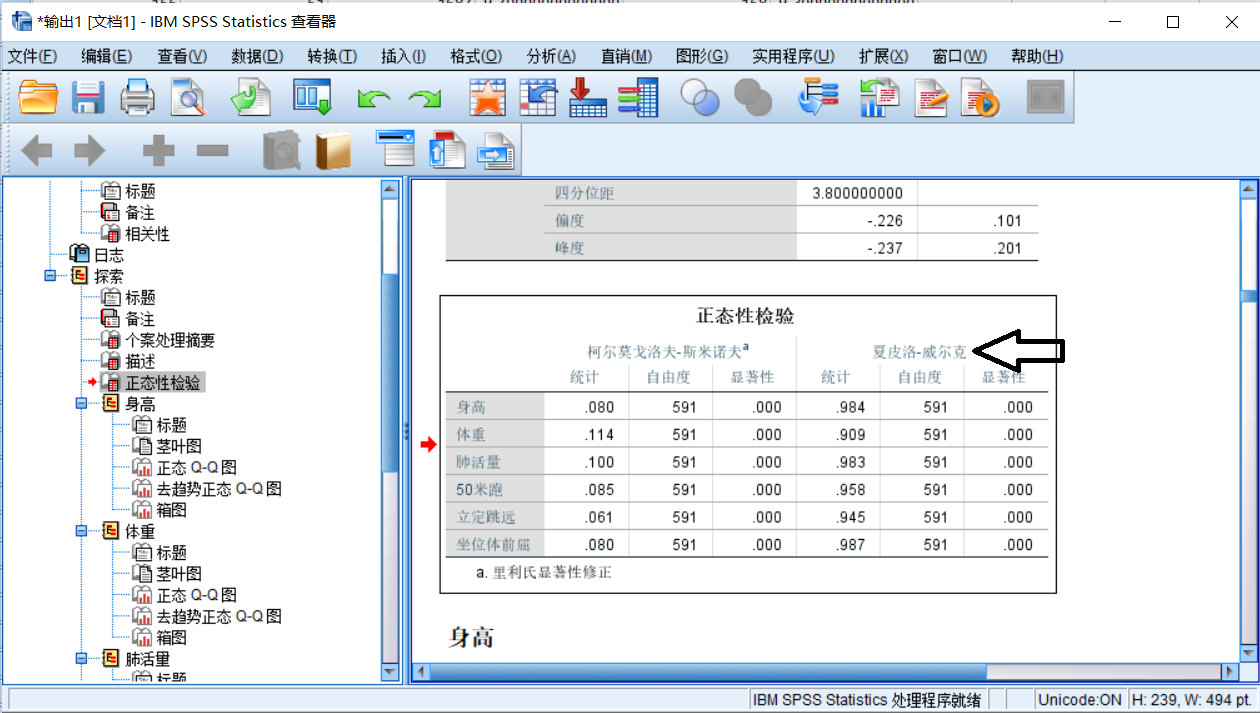(3)皮尔逊相关系数
1.总体皮尔逊Person相关系数
$\rho_{XY}=\dfrac{Cov(X,Y)}{\sigma_x\sigma_y}$
这里$\sigma_x=\sqrt{\dfrac{\displaystyle\sum_{i=1}^{n}(X_i-E(X))^2}{n}}$与$\sigma_y=\sqrt{\dfrac{\displaystyle\sum_{i=1}^{n}(Y_i-E(Y))^2}{n}}$分别是$X$总体与$Y$总体的方差。

$Cov(X,Y)=\dfrac{\displaystyle\sum_{i=1}^{n}(X_i-E(X))(Y_i-E(Y))}{n}$为总体的协方差。
2.样本皮尔逊Person相关系数
$r_{XY}=\dfrac{Cov(X,Y)}{S_xS_y}$
这里$S_x=\sqrt{\dfrac{\displaystyle\sum_{i=1}^{n}(X_i-\overline{X})^2}{n-1}}$与$S_y=\sqrt{\dfrac{\displaystyle\sum_{i=1}^{n}(Y_i-\overline{Y})^2}{n-1}}$分别是$X$样本与$Y$样本的方差。

$Cov(X,Y)=\dfrac{\displaystyle\sum_{i=1}^{n}(X_i-\overline{X})(Y_i-\overline{Y})}{n-1}$为样本的协方差。
3.使用皮尔逊Person相关系数的注意事项

先要判断变量是否大致满足线性关系，可以采用画散点图的方式。当两个变量本来就大致满足线性的关系时，皮尔逊相关系数的绝对值越大，两个变量的相关性越强，但是如果本来就不满足线性关系，就算皮尔逊系数很大也没有意义。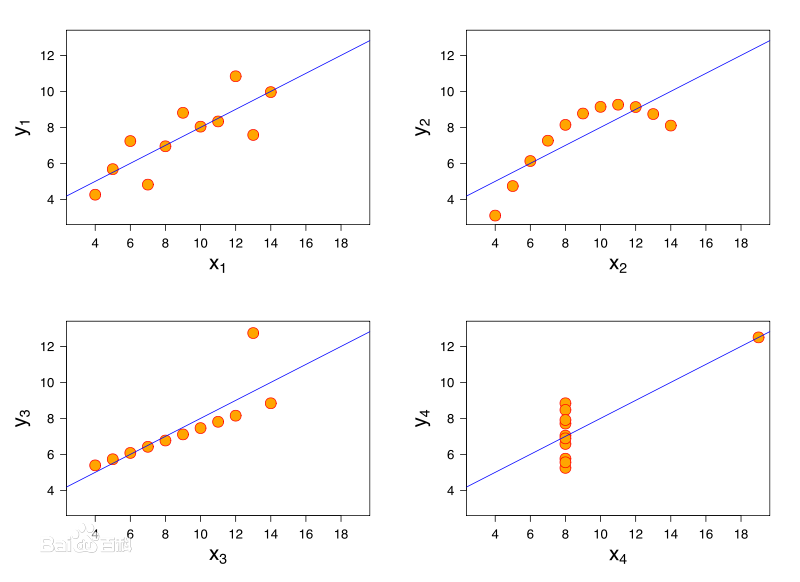上面的几个图的皮尔逊相关系数都为0.816，但是明显小样本对最终结果产生巨大的影响。

如果计算出皮尔逊系数为0，不代表两个变量之间不存在关系。比如下面的温度-冰糕之间必然存在相关性，但是不是线性关系。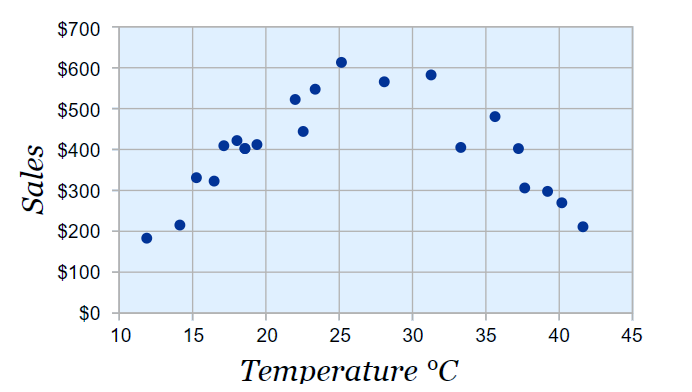可以使用 SPSS 作出散点图先判断是否具有一定的线性关系。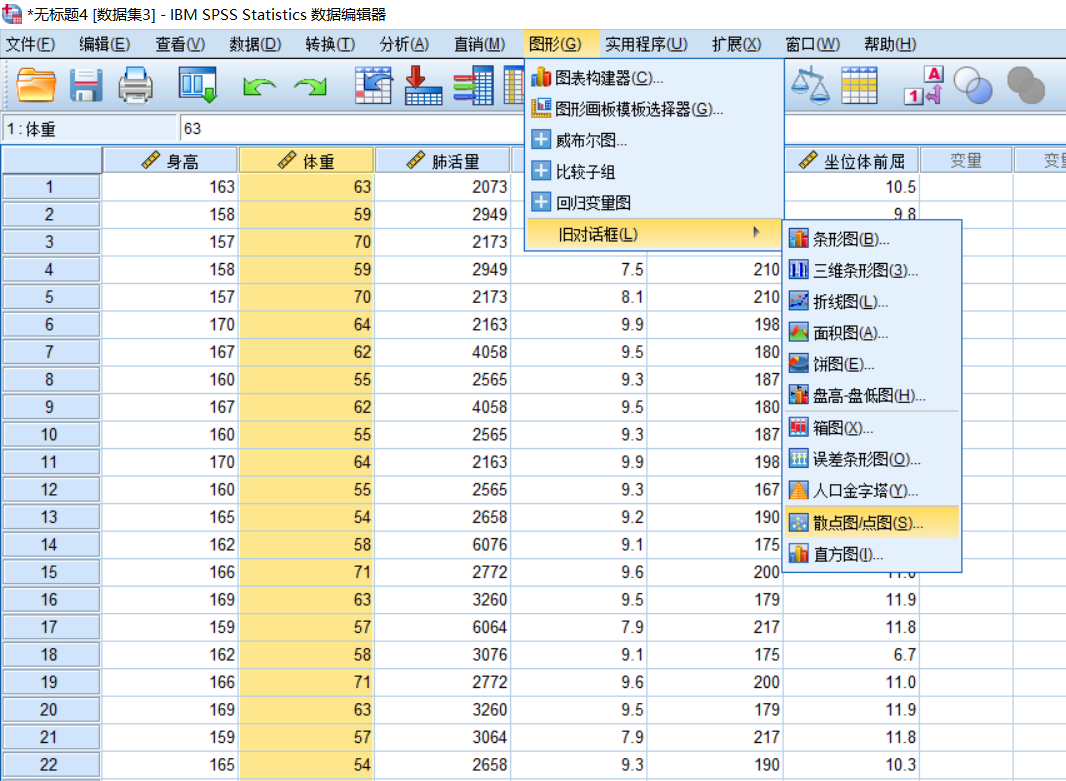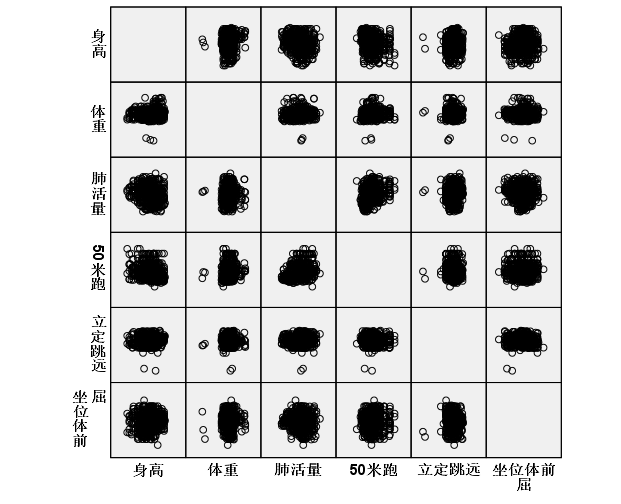4.使用matlab计算person系数
$①$使用matlab计算
corrcoef

R=corrcoef(A)：返回$A$的相关系数矩阵，其中$A$的列表示随机变量(指标，例如身高、体重等),行表示观测值(样本，例如每一个人的身高、体重等)。
R=corrcoef(A,B)：返回两个变量$A，B$向量之间的相关系数。

$②$使用Excel计算与美化相关系数表

在数据分析中选取相关系数的计算。
使用色阶图进行美化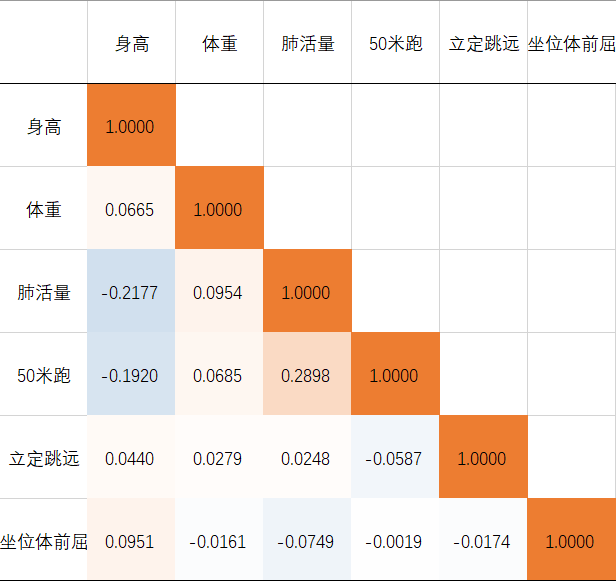(3)对皮尔逊相关系数进行假设检验
1.可以进行假设检验的前提

实验数据通常假设是成对的来自于正态分布的总体。(一般情况下很难满足)。
实验数据之间的差距不能太大，异常值对检验结果影响很大。
每个样本之间是独立抽样的。

2.进行正态分布检验
3.流程
⭐️目的是验证计算得出的皮尔逊相关系数是否与0有显著的差异。

step 1  提出原假设与备择假设,$H_0:r=0,H_1:r\not=0$。

step 2 构造检验统计量
$r\sqrt{\dfrac{n-2}{1-r^2}}\sim t(n-2)$服从自由度为2的$t$分布

step 3 计算出检验值(代入数据)得到 $t^*$。

step 4 使用 $p$ 检验

计算 $p$ 值
$p=2\times(1-\int_{-\infty}^{t^*}t(x)dx)$

step 5 结果说明

$p<0.01$：在 $99\%$ 的置信水平上拒绝原假设。
$0.01：在 $99\%$ 的置信水平上无法拒绝原假设，但在  $95\%$ 的水平上可以拒绝原假设。
$0.05：在 $95\%$ 的置信水平上无法拒绝原假设，但在  $90\%$ 的水平上可以拒绝原假设。

显著性标记: $a,a^*,a^{**}$,** 表示在0.01级别（双尾）的相关性显著，* 表示在0.05级别（双尾）的相关性显著。

matlab相关:

tpdf(x,n)：$x$取一系列连续的值，可以做出自由度为$n$的$t$分布图像。
tcdf(t,n)：是自由度为$n$的$t$分布的分布函数。$t$取一个固定的值$t_c$,可以计算出$T\le t_c$的概率。
tinv(p,n)：自由度为$n$的$t$分布下对应概率为$p$的点的值。

(4)斯皮尔曼(sperman)相关系数
1. 斯皮尔曼相关系数的第一种定义

$X$,$Y$ 为两组数据，其斯皮尔曼(等级)相关系数：
$r_s=1-\dfrac{6\displaystyle\sum_{i=1}^{n}d_i^2}{n(n^2-1)}$ $d_i$ 为 $X_i$ 与 $Y_i$ 之间的等级差。( $-1 \le r_s \le 1$)。
等级：将所在列的元素排序后，这个数所在的位置。
有数值相同，将它们所在位置取算术平均。例如对下面一列数 $90,84,76,71,71,71,69,68,66,64$ 排出的顺序为 $1,2,3,5,5,5,7,8,9,10$ 计算方式为 $\dfrac{4+5+6}{3}$。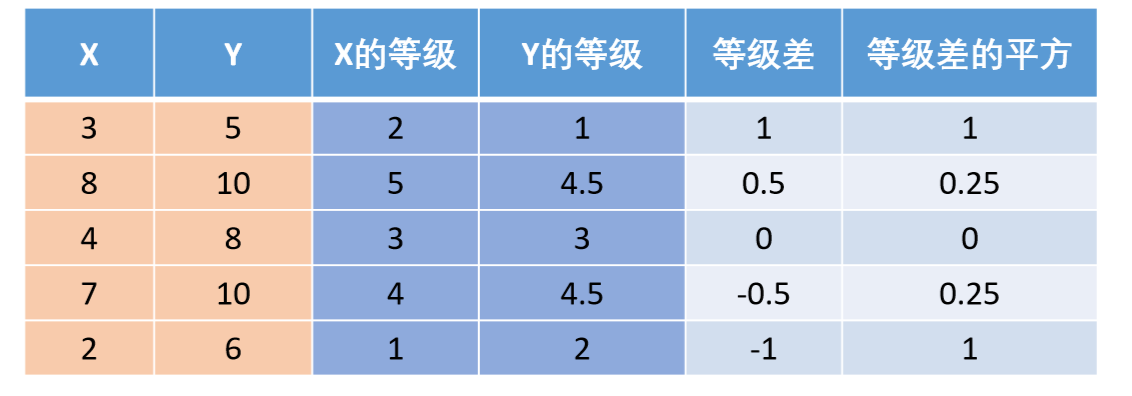2. 斯皮尔曼相关系数的第二种定义

定义为等级之间的皮尔逊相关系数。
按这种定义计算得到的斯皮尔曼相关系数一般与第一种定义下计算出的斯皮尔曼相关系数不同。

3.使用matlab计算斯皮尔曼相关系数

corr(X,Y,'type','Spearman')：其中$X$与$Y$必须是列向量。
corr(X,'type','Spearman')：计算矩阵$X$各列之间的斯皮尔曼相关系数。

(5)斯皮尔曼相关系数的假设检验
$H_0:r_s=0,H_1:r_s\not=0$。
1.小样本情况下$(n\le30)$
直接在临界表中查询即可。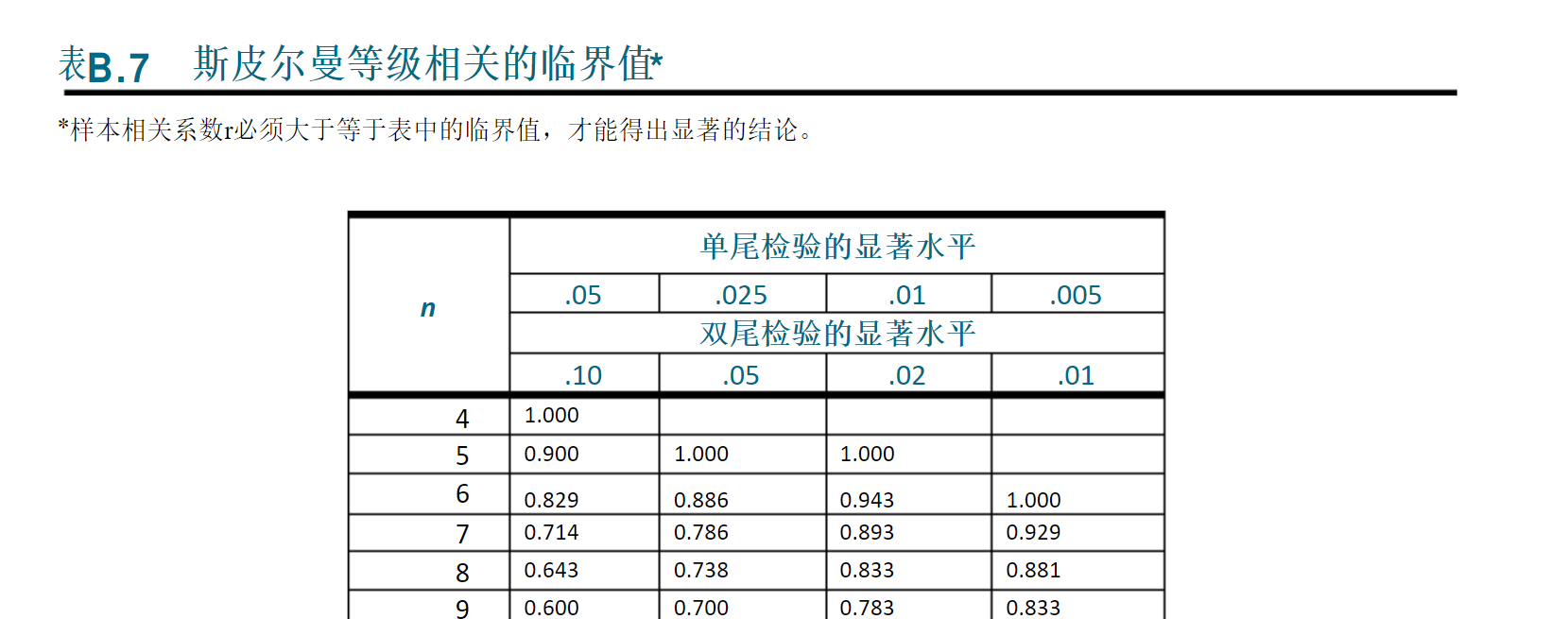2.大样本情况下$(n\ge30)$

统计量 $r_s\sqrt{n-1}\sim N(0,1)$。

计算检验值 $r_s\sqrt{n-1}$。

计算对应的$p$值,再进行判断。

(6)person与spearman对比

连续数据，正态分布，线性关系，最好使用 person。
除此之外都使用 spearman。
两个定序数据之间也只能用spearman。
定序数据：仅仅反映观测对象的等级顺序关系的数据。(例如：优，良，差等)。


展开全文数学建模
• 独立性检验表明的是两者是否有关系，相关性检验说明两者成什么样的关系，无论是否有关系都可以表示出回归方程 1 相关性检验 简单相关系数：度量定量变量间的线性相关关系(非相关性) 复相关系数：因变量与多个自变量...
独立性检验表明的是两者是否有关系，相关性检验说明两者成什么样的关系，无论是否有关系都可以表示出回归方程
1 相关性检验
简单相关系数：度量定量变量间的线性相关关系(非相关性)
复相关系数：因变量与多个自变量之间的关系
偏相关系数：反应矫正其他变量后某一变量与另一变量的相关关系，校正：嘉定其他变量取值均为平均数
典型相关系数：主成分分析后得到性的线性无关的综合指标，通过新的综合指标间的相关系数研究原来的各组指标之间的整体相关性
1.1 皮尔森相关系数
1.1.1 协方差
二维随机变量（X，Y），X与Y之间的协方差定义为$$Cov(X,Y) = E{[X - E(X)][Y - E(Y)]}$$
E为期望
协方差>0,正相关；协方差<0,正相关；协方差=0,不相关
1.1.2 相关系数
相关系数定义:$$Corr(X,Y) = \frac{Cov(X,Y)}{\sqrt{Var(X)}\sqrt{Var(Y)}}=\frac{Cov(X,Y)}{\sigma_x \sigma_y}$$;Var为方差
Corr(X,Y)=1的时候，说明两个随机变量完全正相关；Corr(X,Y)=-1的时候，说明两个随机变量完全负相关；Corr(X,Y)为0，表示X与Y不相关，这里的不相关指的是X与Y没有线性关系
2 独立性检验
2.1 卡方独立性检验Chi-Square Test
在大数据运营场景中，通常用在某个变量(或特征)值是不是和应变量有显著关系。
普通的思维方式，是拿已经知道的结果(硬币是均衡的，没有人做过手脚)，推测出会出现的不同现象的次数。而卡方检验是拿观察到的现象(投正面或反面的次数或者频数)，来判断这个结果(硬币是不是均衡的)。
卡方检验公式为：$$\chi^2 = \sum \frac{(observed - expected)^2}{expected}$$
方法：1.公式求值。2.自由度：假设仅有X1 X2，X1若给定，X2不自由？则自由度为2-1即1。3.置信度：自己设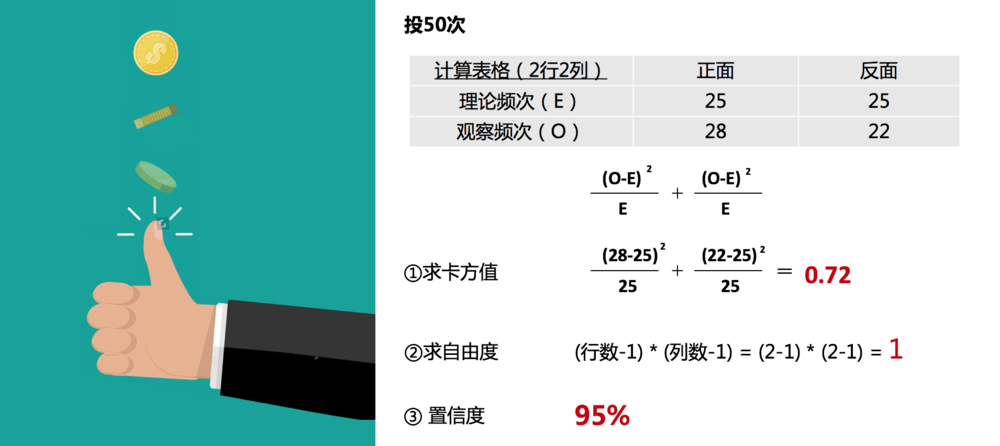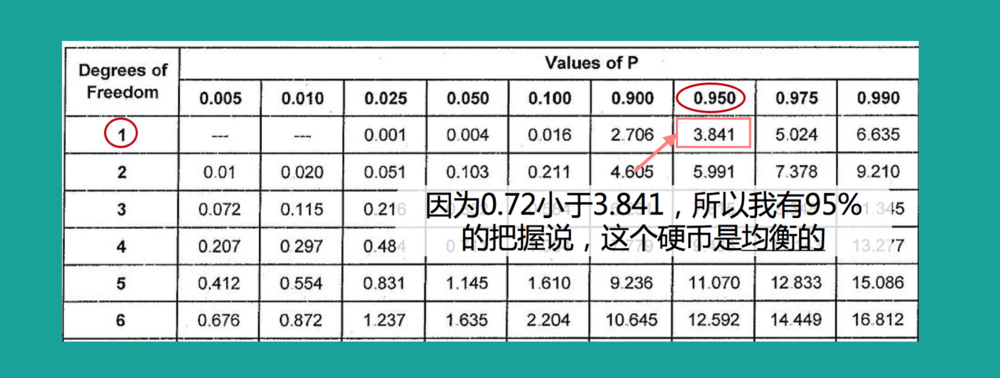拿到这3个信息，去查表，因为0.72小于查表得到的3.841，所以我们得出这个硬币是均衡的结论。

转载于:https://www.cnblogs.com/tillnight1996/p/10703326.html
展开全文• 两个变量之间的相关性检验 1、皮尔森相关系数：它们之间的协方差cov(X,Y)除以它们各自标准差的乘积(σX, σY)。 要判断两个变量之间是否存在相关性：可以采用画散点图的方式，进行直观性的分析。 使用python进行...
两个变量之间的相关性检验
1、皮尔森相关系数：它们之间的协方差cov(X,Y)除以它们各自标准差的乘积(σX, σY)。

要判断两个变量之间是否存在相关性：可以采用画散点图的方式，进行直观性的分析。
使用python进行person检验

import pandas as pd
import numpy as np
import missingno


df = pd.read_csv('./titanic/train.csv')


PassengerId
Survived
Pclass
Name
Sex
Age
SibSp
Parch
Ticket
Fare
Cabin
Embarked

0
1
0
3
Braund, Mr. Owen Harris
male
22.0
1
0
A/5 21171
7.2500
NaN
S

1
2
1
1
Cumings, Mrs. John Bradley (Florence Briggs Th...
female
38.0
1
0
PC 17599
71.2833
C85
C

2
3
1
3
Heikkinen, Miss. Laina
female
26.0
0
0
STON/O2. 3101282
7.9250
NaN
S

3
4
1
1
Futrelle, Mrs. Jacques Heath (Lily May Peel)
female
35.0
1
0
113803
53.1000
C123
S

4
5
0
3
Allen, Mr. William Henry
male
35.0
0
0
373450
8.0500
NaN
S

df.columns

Index(['PassengerId', 'Survived', 'Pclass', 'Name', 'Sex', 'Age', 'SibSp',
'Parch', 'Ticket', 'Fare', 'Cabin', 'Embarked'],
dtype='object')

import seaborn as sns
from matplotlib import pyplot as plt



df = df[['PassengerId','Survived','Pclass','Sex','Age','SibSp','Parch']]
sns.pairplot(df,size = 2.5,diag_kind= 'kde',kind = 'reg')

c:\python39\lib\site-packages\seaborn\axisgrid.py:1969: UserWarning: The size parameter has been renamed to height; please update your code.
warnings.warn(msg, UserWarning)

<seaborn.axisgrid.PairGrid at 0x1e8bb9a7370>

​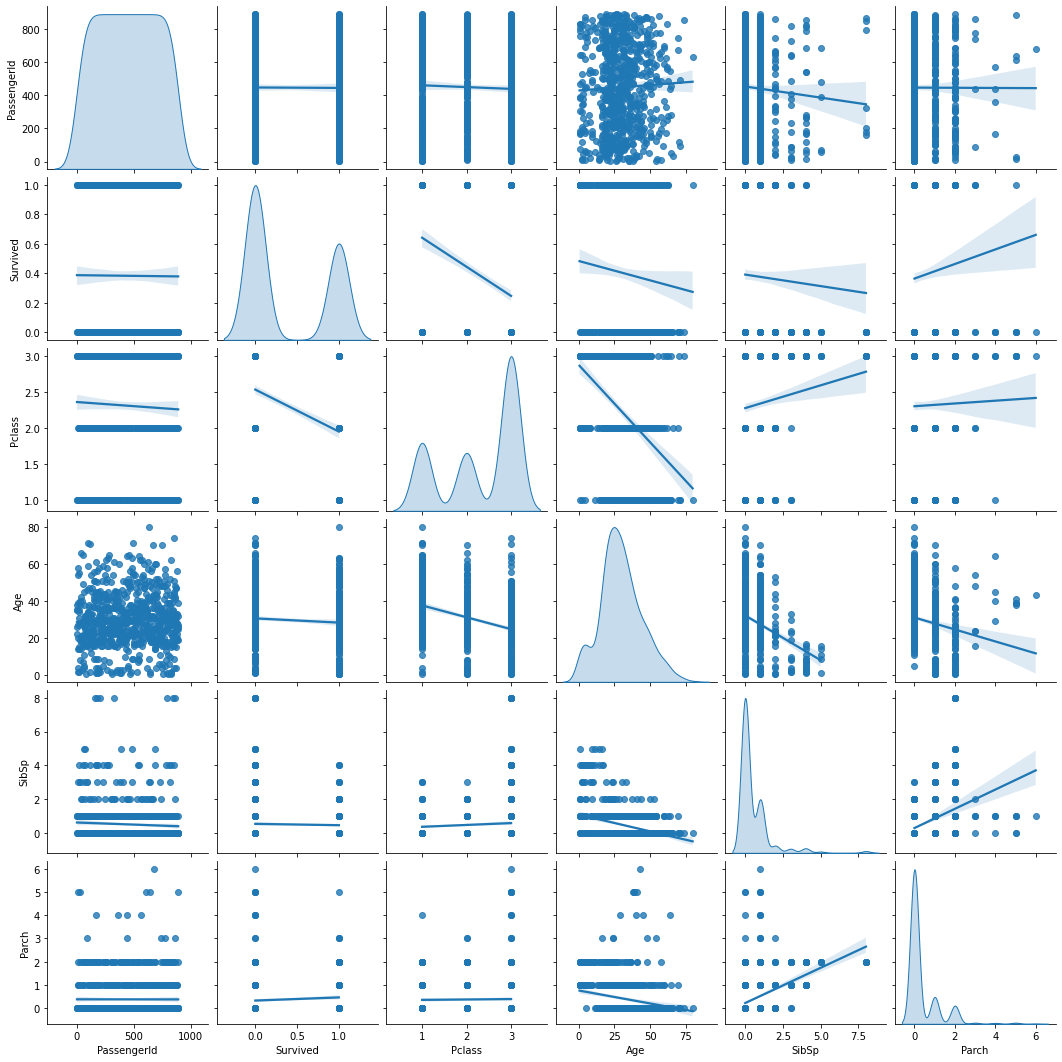​


df.corr()


PassengerId
Survived
Pclass
Age
SibSp
Parch
Fare

PassengerId
1.000000
-0.005007
-0.035144
0.036847
-0.057527
-0.001652
0.012658

Survived
-0.005007
1.000000
-0.338481
-0.077221
-0.035322
0.081629
0.257307

Pclass
-0.035144
-0.338481
1.000000
-0.369226
0.083081
0.018443
-0.549500

Age
0.036847
-0.077221
-0.369226
1.000000
-0.308247
-0.189119
0.096067

SibSp
-0.057527
-0.035322
0.083081
-0.308247
1.000000
0.414838
0.159651

Parch
-0.001652
0.081629
0.018443
-0.189119
0.414838
1.000000
0.216225

Fare
0.012658
0.257307
-0.549500
0.096067
0.159651
0.216225
1.000000

plt.figure(figsize = (12,8))
sns.heatmap(df.corr());

​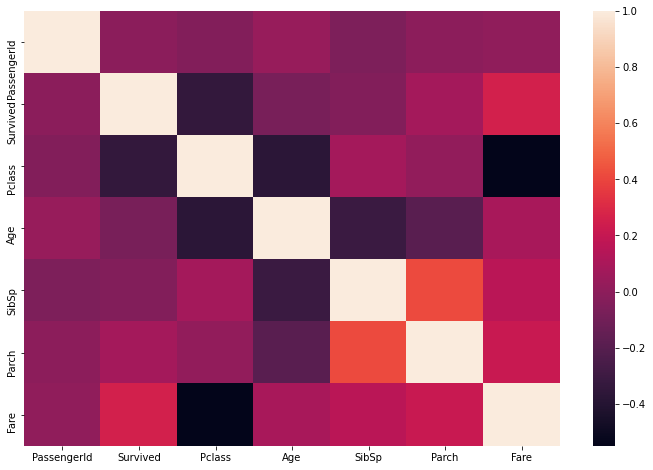​




展开全文python
• 聚类之前要对各指标做相关性检验，对于相关性大的指标可以考虑予以剔除，否则相关性大的指标在分析时会加重他们的权重，影响真实的聚类效果。聚类分析
• 数据分析中的各种相关性检验方法 分清数据类型 要做相关性分析，对于数据首先要认清 数据最基础分为计数资料和计量资料 计数资料：定性资料 性别，舱号，是否生还等 计量资料：定量资料 年龄 选择检验的方式 计量...数据分析
• 数据特征分析技能—— 相关性检验 相关性分析是指对两个或多个具备相关性的变量元素进行分析，从而衡量两个变量因素的相关密切程度 一般常用四种方法： - 画图判断 - pearson（皮尔逊）相关系数 - ...皮尔逊相关系数 斯皮尔曼相关系数
• 论文研究-面板数据空间误差分量模型的空间相关性检验.pdf, 将截面误差分量模型(spatial error components, SEC)扩展至面板数据, 推导其联合检验、边际检验及条件检验, ...
• R语言详解参数检验和非参数检验二、参数检验R语言实现2.1 单样本t检验2.2 独立样本t检验2.3 配对样本t检验2.4方差分析2.5 pearson相关性检验三、非参数检验R语言实现3.1单样本wilcoxon检验3.2 Mann-Whitney检验3.3...数据分析 机器学习 数据挖掘
• 数据特征分析技能—— 相关性检验 相关性分析是指对两个或多个具备相关性的变量元素进行分析，从而衡量两个变量因素的相关密切程度 一般常用四种方法： - 画图判断 - pearson（皮尔逊）相关系数 - sperman（斯皮尔曼...
• 那由样本推及总体的相关性检验该如何做呢？连续变量可以在皮尔逊相关系数里直接做，分类变量怎么搞？且看今日分享。提前预习统计学定类、定序、定距卡方检验U检验、H检验SPSS操作分析-描述统计-交叉表分析-表-定制表...
• 今天做空气质量数据分析时候碰到相关性检验的问题。 问题描述：有两种污染物浓度数据，怎么判断这两种污染物浓度之间是否存在相关性？正相关或负相关，即当A浓度上升时，B的浓度同时上升或下降？（这是相关性的...
• 相关性检验–Spearman秩相关系数和皮尔森相关系数
• 1.6　相关性检验 对于多元数据,讨论变量间是否具有相关关系是很重要的,这里介绍三种相关检验—Pearson相关检验、Spearman相关检验和Kendall相关检验,第一个检验是针对正态数据而言的,而后面两种检验属于秩检验. ...
• 1.6　相关性检验 对于多元数据,讨论变量间是否具有相关关系是很重要的,这里介绍三种相关检验—Pearson相关检验、Spearman相关检验和Kendall相关检验,第一个检验是针对正态数据而言的,而后面两种检验属...
• 1.相关性分析        相关性分析是指对两个或多个具备相关性的变量元素进行分析，从而衡量两个变量因素的相关密切程度。相关性的元素之间需要存在一定的联系或者概率才可以进行...
• 相关性检验之Pearson系数及python实现一、Pearson相关系数皮尔森相关系数是用来反应俩变量之间相似程度的统计量，在机器学习中可以用来计算特征与类别间的相似度，即可判断所提取到的特征和类别是正相关、负相关还是...
• 相关性检验--Spearman秩相关系数和皮尔森相关系数 原文：http://www.cnblogs.com/zhangchaoyang/articles/2631907.html 本文给出两种相关系数，系数越大说明越相关。你可能会参考另一篇博客独立性检验。 皮尔森...概率统计 皮尔森相关系数
• 相关性反应的是变量之间的变换趋势和程度。范围在-1，+1之间，正值为正相关，负值为负相关，在样本数量充足的情况下，0即意味着两个变量是完全独立的。 1.皮尔森系数（peaarson） 皮尔森系数是利用协方差与...
• 本文仅根据自己实际论文情况做的总结。...2、相关性矩阵计算 #读取数据 data=read.csv('D:/论文/撰写阶段/常用/匹配完未计算收益率数据.csv') #计算相关性矩阵 cor_matr = cor(data) 这里碰见几次错误 Error i...数据分析...